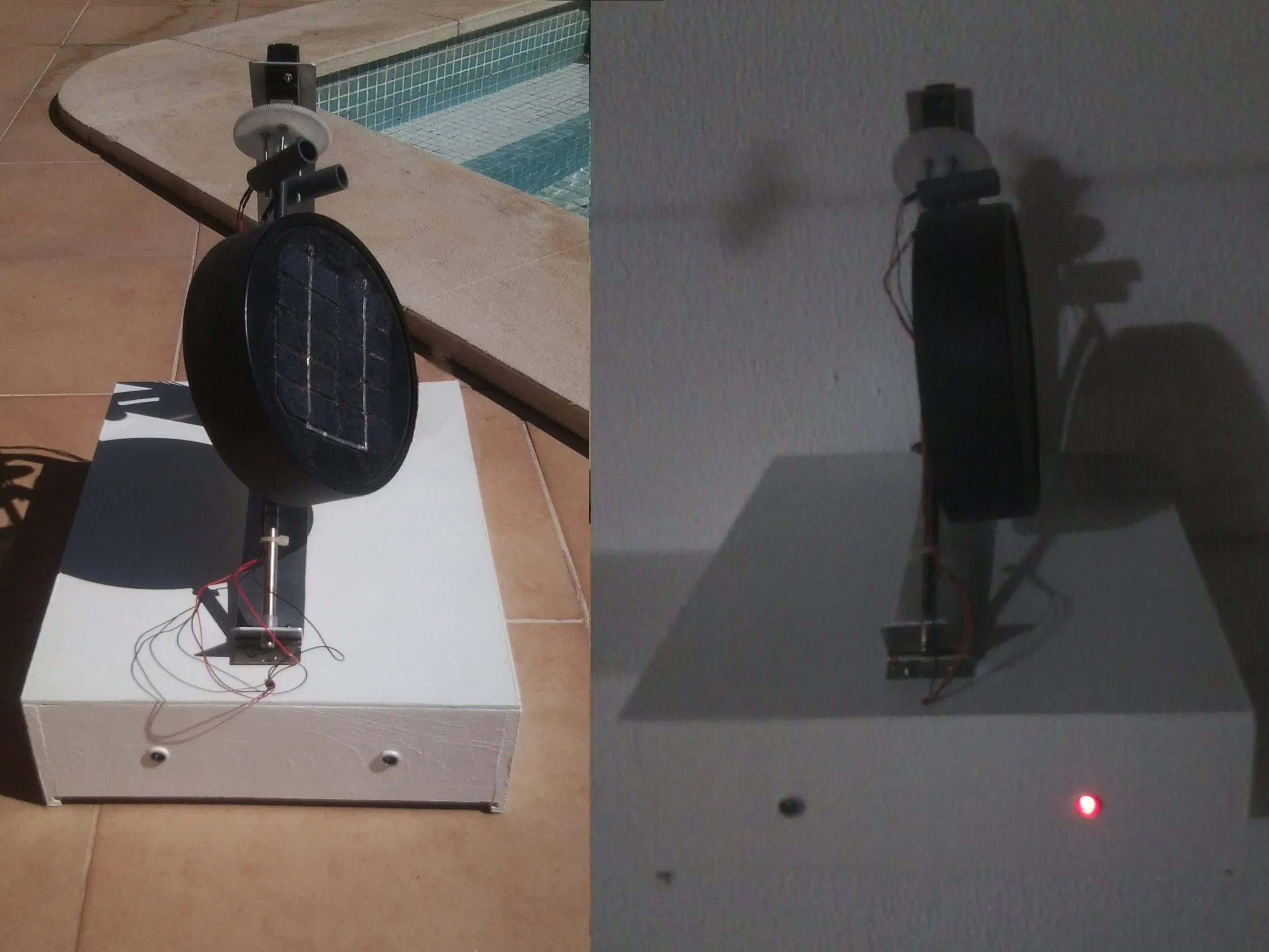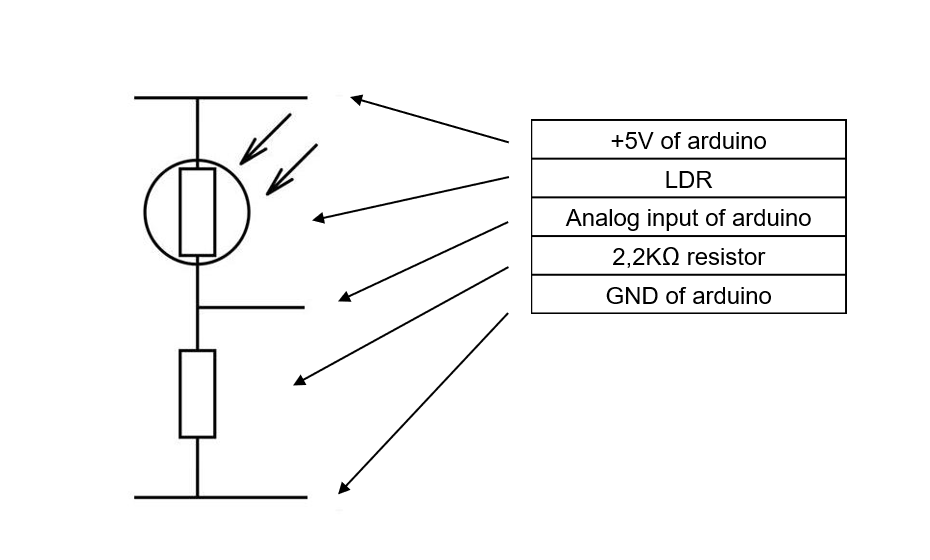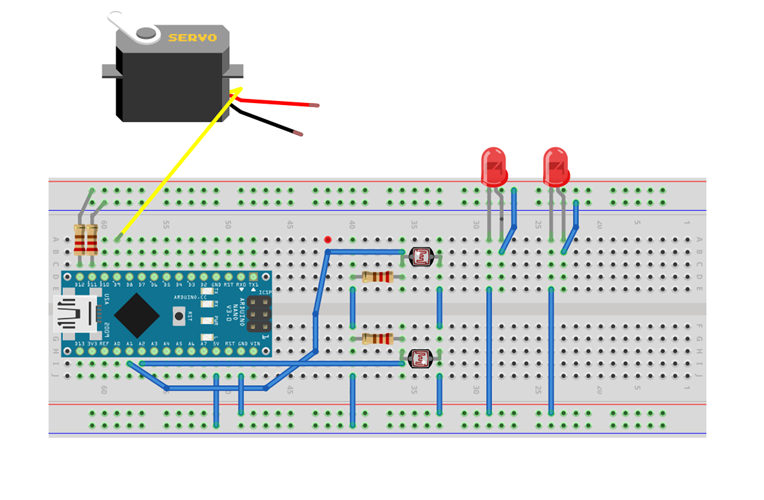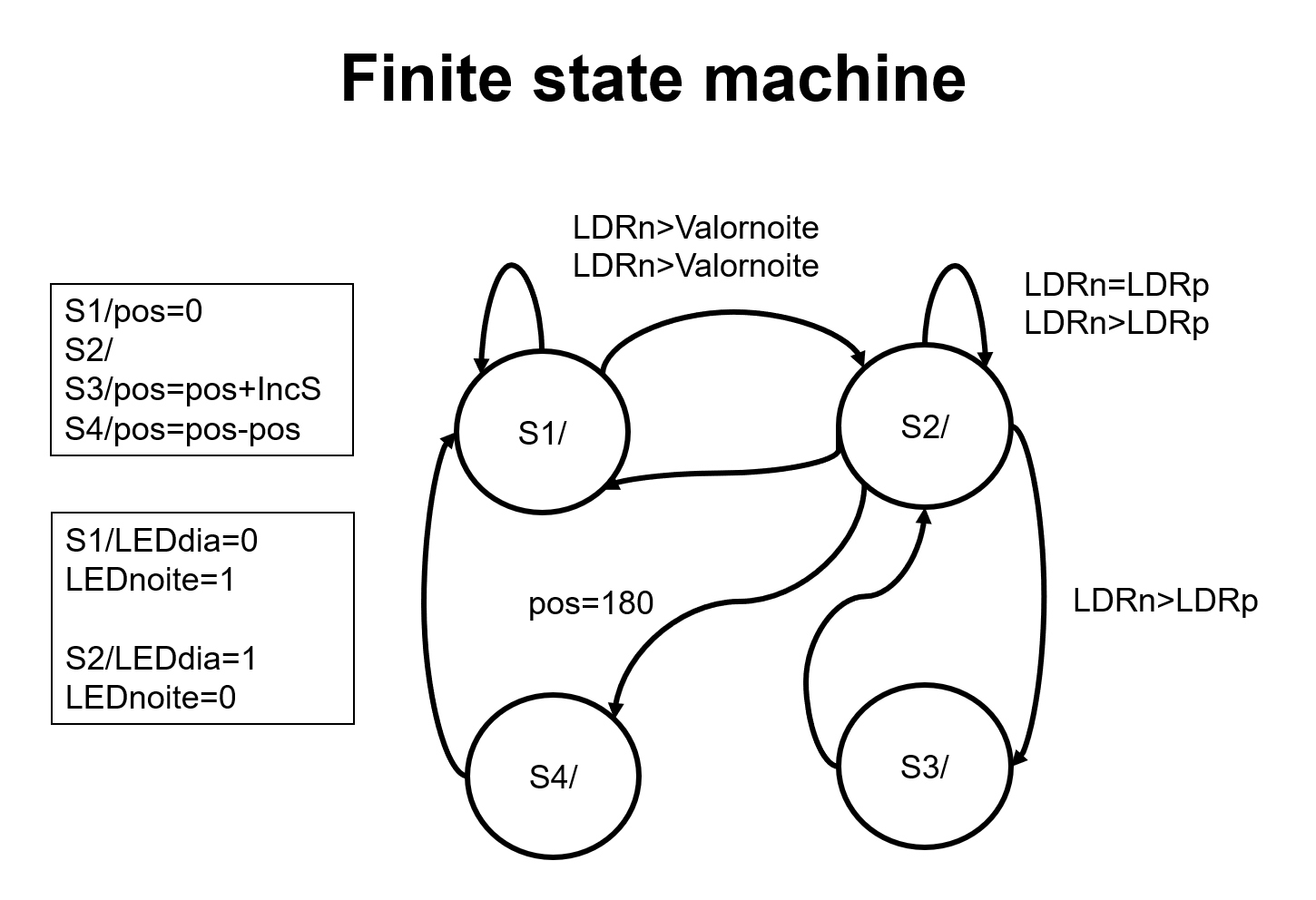Project tutorial# Solar Traker

A system that follows the sun, with a servo connected to an arduino, programmed with a finite state machine in C language.

• 1,577 views
• 7 respects

## Necessary tools and machinesSoldering iron (generic)

1. Objective

This project has the objective of creating a system that increases the solar exposure of a photovoltaic cell, using one axial with two Light Dependent Resistor and one servo conected to an arduino nano, programed with a finite state machine in C language.

2. Testing the components

Servo:

To power the servo it needs an 5V from external power supply, because the arduino nano can not supply enough current. And the sinal to the analog output 9 of the arduino. (Note conect the negative of power supply to GND of arduino) Code for testing the servo:

``````#include <Servo.h>
Servo servo;        // create servo object to control a servo
int pos = 10;       // variable to store the servo position
void setup()
{
Serial.begin(9600);
servo.attach(9);   // attaches the servo on pin 9 to the servo object
servo.write (10);  //write any position from 0 to 180, for exemple 10
}
``````

How to read Light Dependent Resistor, code:

``````const int pinLDRn = A0;   //attaches LDR to analog input on servo
const int pinLDRp = A1;
int LDRn = 0;             //first read at the start
int LDRp = 0;
void setup()
{
Serial.begin(9600)
pinMode(LDRn, INPUT);
pinMode(LDRp, INPUT);
}
void loop()
{
int LDRn;
int LDRp;
Serial.print("LDRn = " );
Serial.println(LDRn);
Serial.print("LDRp = " );
Serial.println(LDRp);
}
``````

To monotorize the reading do Ctrl+Shift+M, while conected to arduino in the software.

## Code

##### FSM_solar_trb2_publicar_net.inoC/C++
```#include <Servo.h>
Servo servo;

const int pinLDRn = A0;
const int pinLDRp = A1;
const int LEDdia = 12;
const int LEDnoite = 11;
int LDRn = 0;
int LDRp = 0;
int pos = 10;
int valornoite = 1000;
int valorfim = 170;
const int IncS = 1;
static int FSM0_state = 1;

void setup()
{
Serial.begin(9600);
servo.attach(9);
servo.write (10);
pinMode(LDRn, INPUT);
pinMode(LDRp, INPUT);
pinMode(LEDdia, OUTPUT);
pinMode(LEDnoite, OUTPUT);
}

void loop()
{
int LDRn;
int LDRp;
int FSM0_e_noite;
int FSM0_e_sub;
int FSM0_e_IncS;
if (LDRn > valornoite && LDRp > valornoite){FSM0_e_noite = 1;}
else FSM0_e_noite = 0;
if (pos > valorfim) {FSM0_e_sub = 1;}
else {FSM0_e_sub = 0;}
if (LDRn > LDRp){FSM0_e_IncS = 1;}
else {FSM0_e_IncS = 0;}
FSM0(LDRn,LDRp,FSM0_e_noite,FSM0_e_sub,FSM0_e_IncS);

//Serial.print("LDRn = " );
//Serial.println(LDRn);
//Serial.print("LDRp = " );
//Serial.println(LDRp);
//delay (500);
}

void FSM0(int LDRn, int LDRp, int FSM0_e_noite, int FSM0_e_sub, int FSM0_e_IncS)
{
FSM0_next_state(LDRn,LDRp, FSM0_e_noite,FSM0_e_sub,FSM0_e_IncS);
FSM0_output();
}

void FSM0_next_state (int LDRn, int LDRp, int FSM0_e_noite, int FSM0_e_sub, int FSM0_e_IncS)
{
switch(FSM0_state)
{
case 1:
if (FSM0_e_noite == 1){FSM0_state = 1;}
else FSM0_state = 2;
break;
case 2:
if (FSM0_e_sub == 1) {FSM0_state = 4;}
else if (FSM0_e_noite == 1){FSM0_state = 4;}
else if (FSM0_e_IncS){FSM0_state = 3;}
else FSM0_state = 2;
break;
case 3:
FSM0_state = 2;
break;
case 4:
FSM0_state = 1;
break;
default:
FSM0_state = 1;
break;
}
Serial.print("FSM0_state = ");
Serial.println(FSM0_state);
}

void FSM0_output()
{
switch(FSM0_state)
{
case 1:
digitalWrite(LEDdia,LOW);
digitalWrite(LEDnoite,HIGH);
pos = 5;
servo.write (pos);
break;
case 2:
digitalWrite(LEDnoite,LOW);
digitalWrite(LEDdia,HIGH);
break;
case 3:
pos = pos+IncS;
servo.write (pos);
break;
case 4:
pos = pos-pos;
servo.write (pos);
break;
default:
break;
}
Serial.print("pos = ");
Serial.println(pos);
}
```

## SchematicsMay 11, 2018

#### Members who respect this project

See similar projects
you might like

#### Dual Axis Solar Tracker Panel with Auto and Manual Mode

Project tutorial by Giannis Arvanitakis

• 17,997 views
• 63 respects

#### Solar Panel Sun Tracker - Phone Charger

Project tutorial by FIELDING

• 43,987 views
• 172 respects

#### Integrated Solar ChargeController, Inverter, PowerBank, Lamp

Project tutorial by Shahariar

• 12,053 views
• 42 respects

Project tutorial by Kutluhan Aktar

• 4,174 views
• 16 respects

#### Light Intensity and Solar Panel Energy Detector

Project tutorial by Kutluhan Aktar

• 3,252 views